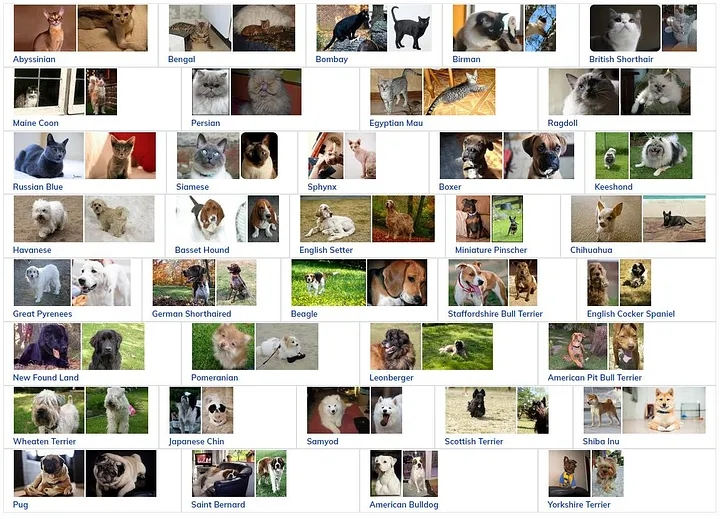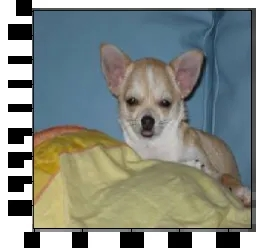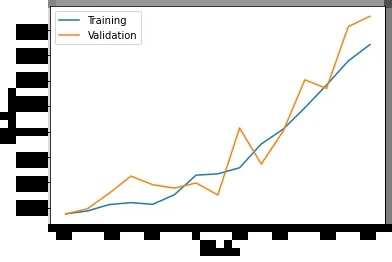400-808-2006# 使用Tensorflow构建您的第一个CNNimport pandas as pd

import glob

import numpy as np

import matplotlib.pyplot as plt

import tensorflow as tf

from tensorflow import keras

from keras.preprocessing.image import ImageDataGenerator12种猫和25种狗。我们将遵循的策略是使用所有的狗品种作为狗的图像，并使用所有的猫品种作为猫的图像。我们不会考虑品种，因为我们想建立一个二进制分类器。

print('There are {} images in the dataset'.format(len(glob.glob('images/*.jpg'))))

CATS = ['Abyssinian', 'Bengal', 'Birman', 'Bombay', 'British_Shorthair', 'Egyptian_Mau', 'Maine_Coon', 'Persian', 'Ragdoll', 'Russian_Blue', 'Siamese', 'Sphynx']

cats_images = []

dogs_images = []

for img in glob.glob('images/*.jpg'):

if any(cat in img for cat in CATS):

cats_images.append(img)

else:

dogs_images.append(img)

print('There are {} images of cats'.format(len(cats_images)))

print('There are {} images of dogs'.format(len(dogs_images)))

#shuffle the lists

np.random.shuffle(cats_images)

np.random.shuffle(dogs_images)

#split the data into train, validation and test sets

train_d, val_d, test_d = np.split(dogs_images, [int(len(dogs_images)*0.7), int(len(dogs_images)*0.8)])

train_c, val_c, test_c = np.split(cats_images, [int(len(cats_images)*0.7), int(len(cats_images)*0.8)])

train_dog_df = pd.DataFrame({'image':train_d, 'label':'dog'})

val_dog_df = pd.DataFrame({'image':val_d, 'label':'dog'})

test_dog_df = pd.DataFrame({'image':test_d, 'label':'dog'})

train_cat_df = pd.DataFrame({'image':train_c, 'label':'cat'})

val_cat_df = pd.DataFrame({'image':val_c, 'label':'cat'})

test_cat_df = pd.DataFrame({'image':test_c, 'label':'cat'})

train_df = pd.concat([train_dog_df, train_cat_df])

val_df = pd.concat([val_dog_df, val_cat_df])

test_df = pd.concat([test_dog_df, test_cat_df])

print('There are {} images for training'.format(len(train_df)))

print('There are {} images for validation'.format(len(val_df)))

print('There are {} images for testing'.format(len(test_df)))

BATCH_SIZE = 32

IMG_HEIGHT = 224

IMG_WIDTH = 224

#create the ImageDataGenerator object and rescale the images

trainGenerator = ImageDataGenerator(rescale=1./255.)

valGenerator = ImageDataGenerator(rescale=1./255.)

testGenerator = ImageDataGenerator(rescale=1./255.)

#convert them into a dataset

trainDataset = trainGenerator.flow_from_dataframe(

dataframe=train_df,

class_mode="binary",

x_col="image",

y_col="label",

batch_size=BATCH_SIZE,

seed=42,

shuffle=True,

target_size=(IMG_HEIGHT,IMG_WIDTH) #set the height and width of the images

)

valDataset = valGenerator.flow_from_dataframe(

dataframe=val_df,

class_mode='binary',

x_col="image",

y_col="label",

batch_size=BATCH_SIZE,

seed=42,

shuffle=True,

target_size=(IMG_HEIGHT,IMG_WIDTH)

)

testDataset = testGenerator.flow_from_dataframe(

dataframe=test_df,

class_mode='binary',

x_col="image",

y_col="label",

batch_size=BATCH_SIZE,

seed=42,

shuffle=True,

target_size=(IMG_HEIGHT,IMG_WIDTH)

)

images, labels = next(iter(testDataset))

print('Batch shape: ', images.shape)

print('Label shape: ', labels.shape)

plt.imshow(images)

print('Label: ', labels)model = keras.Sequential([

keras.layers.InputLayer(input_shape=(IMG_HEIGHT, IMG_WIDTH, 3)),

keras.layers.Conv2D(64, (3, 3), activation='relu'),

keras.layers.MaxPooling2D((2, 2)),

keras.layers.Conv2D(128, (3, 3), activation='relu'),

keras.layers.MaxPooling2D((2, 2)),

keras.layers.Conv2D(256, (3, 3), activation='relu'),

keras.layers.MaxPooling2D((2, 2)),

keras.layers.Conv2D(512, (3, 3), activation='relu'),

keras.layers.GlobalAveragePooling2D(),

keras.layers.Dense(1, activation='sigmoid')

])

epochs=15

history = model.fit(trainDataset, epochs=epochs, validation_data=(valDataset))

plt.plot(history.history['accuracy'])

plt.plot(history.history['val_accuracy'])

plt.xlabel('Epoch')

plt.ylabel('Accuracy')

plt.legend(['Training', 'Validation'])

plt.show()loss, acc = model.evaluate(testDataset)

print('Loss:', loss)

print('Accuracy:', acc)def preprocess(image):

img_resize = tf.image.resize(image, [IMG_HEIGHT, IMG_WIDTH])

img_norm = img_resize / 255

return img_norm

img = tf.reshape(img, (-1, IMG_HEIGHT, IMG_HEIGHT, 3))

img = preprocess(img)

model.predict(img)

## 近期开班

• ### 【全国统一报名热线：400-808-2006】 蒋老师：13260025235 （微信同号） QQ: 2210585665 倪老师：18701378400 （微信同号） QQ：1658122838 方老师：13910781835 （微信同号） QQ：1808273142

•扫码加老师微信
•微信服务号